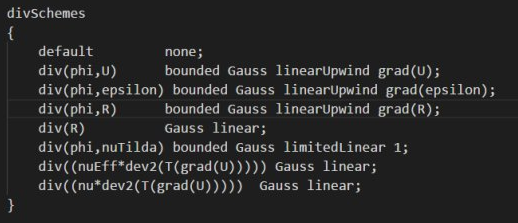# 请问有人见过divSchemes字典中的div((nu * dev2(T(grad(U)))))关键字吗

• 亲爱的Foamers,今天我在博客中看到一个这样的示例, 如图所示，其中div((nuEff * dev2(T(grad(U)))))关键字在湍流算例中都有，但是div((nu * dev2(T(grad(U)))))关键字我在其他算例中都没见过，请问这个算例中的该关键字表示什么意思？还是说这个关键字楼主写错了？是一个无效指令？看他最后结果输出挺好的，，对此疑问，期待你们的回复，谢谢！• div((nuEff*dev2(T(grad(U))))) 这是一个比较长的关键词，根本原因是程序里面需要计算div((nuEff*dev2(T(grad(U)))))，在计算的时候需要指定数值格式。相对应的就需要用户指定。
div((nu*dev2(T(grad(U)))))也是一个关键词。OpenFOAM里面不一定所有的关键词都有用。二者意义大体意义，区别就是一个是nuEff一个是nu，前者调用的是管理有效年度，后者是层流粘度。为什么我能看出来nuEff是有效粘度呢？是因为nu表示粘度，Eff表示effective

• template<class BasicTurbulenceModel>
Foam::tmp<Foam::fvVectorMatrix>
Foam::linearViscousStress<BasicTurbulenceModel>::divDevRhoReff
(
volVectorField& U
) const
{
return
(
- fvc::div((this->alpha_*this->rho_*this->nuEff())*dev2(T(fvc::grad(U))))
- fvm::laplacian(this->alpha_*this->rho_*this->nuEff(), U)
);
}